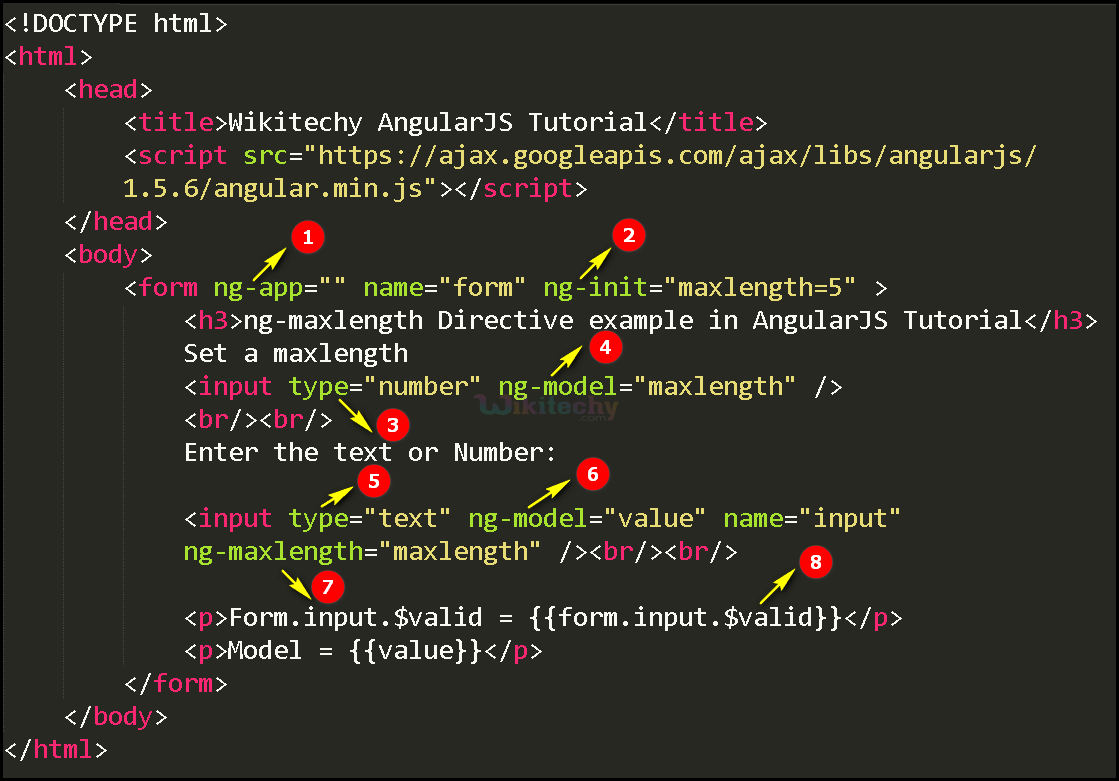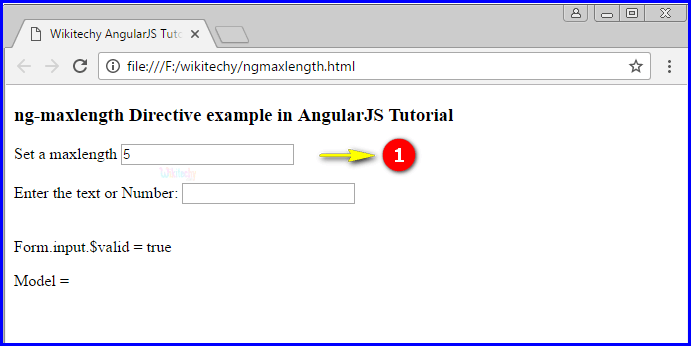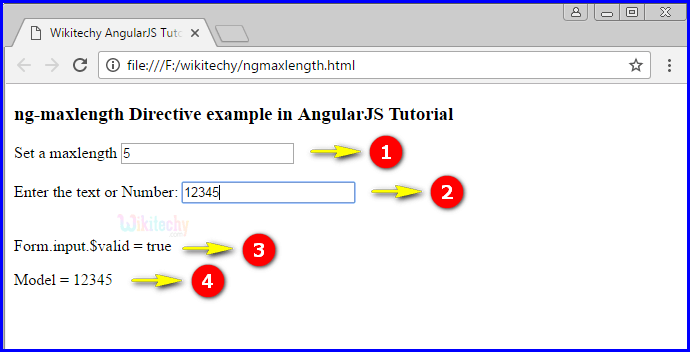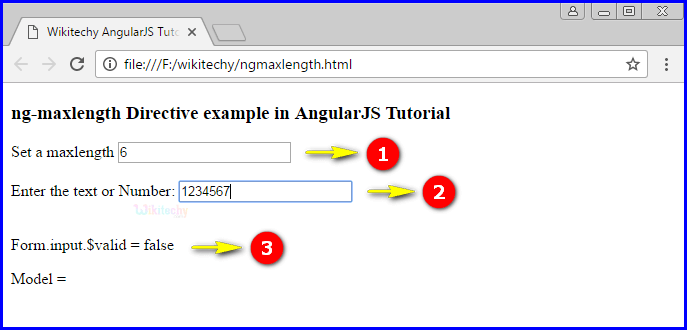# AngularJS ngMaxlength Directive

• The ng-maxlength directive in AngularJS used to restrict an input field with some limitation for the input fields.
• This directive defines the maximum number of characters allowed inside the input field.
• The ng-maxlength directive will not stop the users from typing more than the restricted number of characters, but the form will be invalid (i.e. form. \$valid= false ).
• It is mainly used for text-based input controls but also applied for custom text-based controls.

## Parameter value for ng-maxlength directive in AngularJS:

Value Description
number Denotes the maximum number of characters legal for the input field.

## Using ng-maxlength directive in AngularJS

• Here ng-maxlength directive specifies the maximum number of characters allowed inside the input field.

## Code Explanation for ng-maxlength directive in AngularJS:1. The ng-app specifies the root element (e.g. <body> or <html> or <div> tags) to define AngularJS application.
2. The ng-init is used to initialize the values for an application.(here the value 5 is mentioned for “maxlength”)
3. “number” is declare the type value of the <input> tag.
4. The ng-model bind an input field value to AngularJS application variable (“maxlength”).
5. The “text” is declare the type value of the <input> tag.
6. The ng-model bind an input field value to AngularJS application variable (“value”).
7. The ng-maxlength directive used for specifies the maximum number of characters allowed in the input field. Here “maxlength” is declare the ng-maxlength value of the <input> tag.
8. Form.input.\$valid to checks the valid maxlength format or not. If the specified maxlength is correct and the output displays true otherwise false.

## Sample Output for ng-maxlength directive in AngularJS:1. The output displays the default value of maxlength as “5”.1. The output displays the default value of maxlength as “5”.
2. If the user type the number in the inputfield.
3. The output displays the true because the maxlength value is 5.
4. The output displays the model as “12345”.1. If the user type the maxlength value is 6.
2. If the user type the number in the inputfield.
3. The output displays the false because the maxlength value is 6 but the user type the number in the input field as “1234567”.

## Tips and Notes

• The ngMaxlength does not set the maxlength attribute.
• The ngMaxlength directive must be an expression, while the maxlength attribute value must be interpolated.